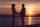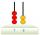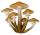Three friends

Peter is eight times older than Rado, Rado is 3 times younger than Joseph. Together they have 120 years. Determine how many are each of them.

Result

P =  80
R =  10
J =  30

Solution:

P=8R
R = J/3
P+R+J= 120

P-8R = 0
J-3R = 0
J+P+R = 120

J = 30
P = 80
R = 10

Calculated by our linear equations calculator.

Leave us a comment of this math problem and its solution (i.e. if it is still somewhat unclear...):Be the first to comment!To solve this verbal math problem are needed these knowledge from mathematics:

Do you have a linear equation or system of equations and looking for its solution? Or do you have quadratic equation?

Next similar math problems:

1. SummerjobThree students participated in the summerjob. Altogether they earn 1780, -. Peter got a third less than John and Paul got 100,- more than Peter. How much got every one of them?
2. Father and daughterWhen I was 11 my father was 35 year old. Now, father has three times older then me. How old is she?
3. Three figures - numbersThe sum of three numbers, if each is 10% larger than the previous one, is 662. Determine the figures.
4. Every day 7 pagesAdelka reads the book every day 7 pages. When she reads one more page a day she will read it three days earlier. How long will Adelka read a book? How much does a book of pages have?
5. MeatHalf a kilogram of pork and three-quarters of a kilogram of beef cost total 5.1 USD. One quarter of a kilogram of beef and 1 kilogram of pork cost 4.9 USD. Determine the price of 1 kg of each type of meat.
6. Time passing6 years ago, Marcela's mother was two times older than her and two times younger than her father. When Marcela is 36, she will be twice as young as her father. How old are Marcela, her father, and mother now?
7. Mom and daughterMother is 39 years old. Her daughter is 15 years. For many years will mother be four times older than the daughter?
8. PairThey have together 39 years - he has 2 times as she was when he was equal as she has today ....
9. ClassroomThere are eighty more girls in the class than boys. Boys are 40 percent and girls are 60 percent. How many are boys and how many girls?
10. Two agesGabrielle is 9 years younger than Mikhail. The sum of their ages is 87. What is Mikhail's age?
11. Boys and girlsThere are 48 children in the sports club, boys are 10 more than girls. How many girls go to the club?
12. MushroomsFor five days, we have collected 410 mushrooms. Interestingly every day we have collected 10 mushrooms more than the preceding day. How many mushrooms we have collected during 4th day?
13. BlackberriesDaniel, Jolana and Stano collected together 34 blackberries. Daniel collected 8 blackberries more than Jolana, Jolana 4 more than Stano. Determine the number blackberries each collected .
14. Two trainsThere were 159 freight wagons on the railway station creating 2 trains. One had 15 more wagons than the other. How many wagons did each train have?
15. Equations - simpleSolve system of linear equations: x-2y=6 3x+2y=4
16. Rabbits 3Viju has 40 chickens and rabbits. If in all there are 90 legs. How many rabbits are there with Viju?
17. Simple equationSolve for x: 3(x + 2) = x - 18